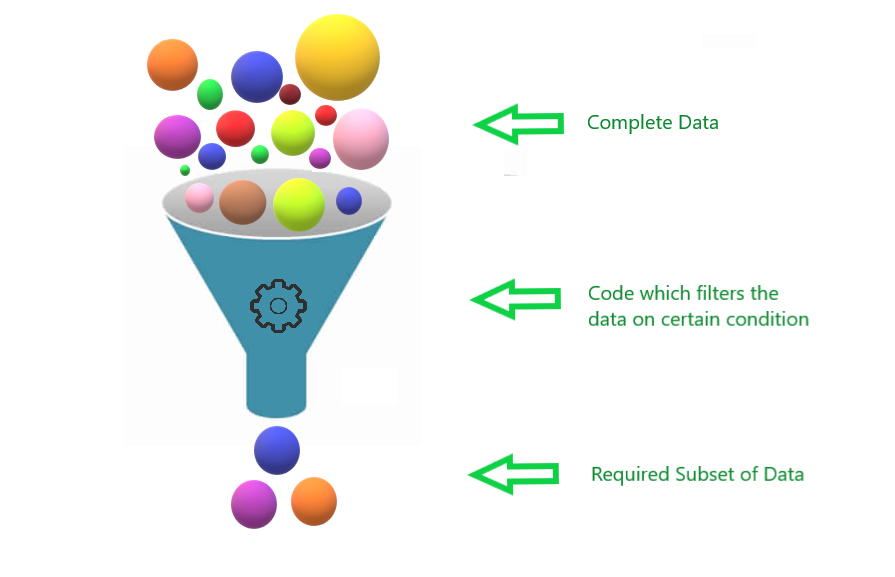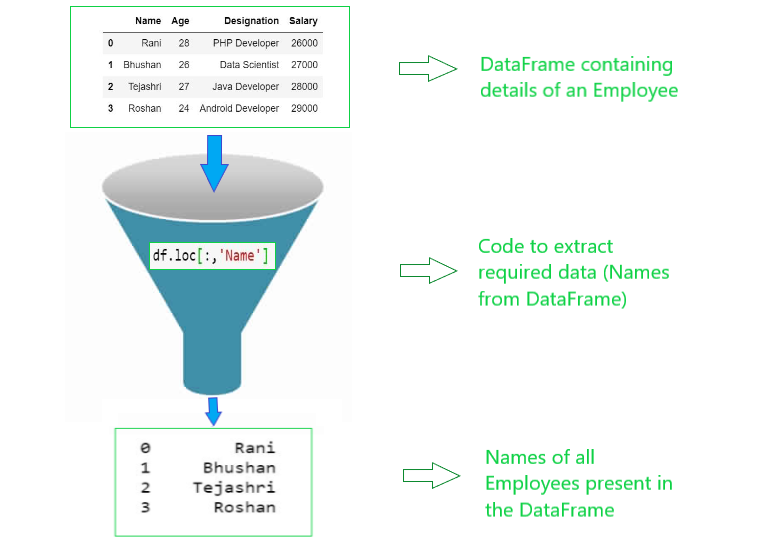# How to Filter rows of DataFrame in Python?

In this article, we will study how we can apply various techniques to filter rows of DataFrame with Python. For this task, we need to first understand the concept of  “Filter”.

What is meant by Filtering the Data?

Data Filtering refers to the process of extracting the required data or a smaller subset of data from the entire Dataset. Filtering the data using Code is similar to people slowly exiting a movie theater after a show ends. This is an example of a time when they filter out of the theatre.

Hence, the filter is used for extracting data that we need.## Python program to filter rows of DataFrame

Let us now look at various techniques used to filter rows of Dataframe using Python.

STEP 1: Import Pandas Library

Pandas is a library written for Python. Pandas provide numerous tools for data analysis and it is a completely open-source library. Here we use Pandas because it provides a unique method to retrieve rows from a data frame. Following line imports pandas:

`import pandas as pd`

STEP 2: Create Sequence

Create Sequence to store the data. Here we have created Dictionary. Details are stored in Dictionary.

```details = {'Name':['Rani','Bhushan','Tejashri','Roshan'],
'Age':[28,26,27,24],'Designation':['PHP Developer',
'Data Scientist','Java Developer','Android Developer'],
'Salary':[26000,27000,28000,29000]}```

STEP 3: Convert Sequence to DataFrame

Convert the above sequence into DataFrame by using Pandas library.

```df = pd.DataFrame(details)
print(df)```

OUTPUT

`Name`
`Age`
`Designation`
`Salary`
`0`
`Rani`
`28`
`PHP Developer`
`26000`
`1`
`Bhushan`
`26`
`Data Scientist`
`27000`
`2`
`Tejashri`
`27`
`Java Developer`
`28000`
`3`
`Roshan`
`24`
`Android Developer`
`29000`

## I) Filter using DataFrame.loc

DataFrame.loc is used to access a group of rows and columns. Hence, using this we can extract required data from rows and columns. Let’s look at some examples by which we will understand exactly how DataFrame.loc works.

Example (i): Here, 0 is the row and ‘Name’ is the column. It will extract data from “0”th row and “Name” column.

`df.loc[0,'Name']`

OUTPUT

‘Rani’

Example (ii): Here, ‘:’ means all rows and ‘Name’ is the column. It will extract data from all the rows and “Name” column.

`df.loc[:,'Name']`

OUTPUT

```0        Rani
1     Bhushan
2    Tejashri
3      Roshan```

Example (iii): Here, 0 is the row and ‘:’ means all columns. It will extract data from”0″th row and all columns.

`df.loc[0,:]`
```Name                    Rani
Age                       28
Designation    PHP Developer
Salary                 26000
```

If we want to retrieve certain rows of the file, we can use DataFrame.head(). DataFrame.head() returns top 5 elements from the sequence. No parameter is passed to .head(). However, it can also take parameters. DataFrame.head(8) will return the top 8 elements from the sequence.

`df.head()`

OUTPUT

`Name`
`Age`
`Designation`
`Salary`
`0`
`Rani`
`28`
`PHP Developer`
`26000`
`1`
`Bhushan`
`26`
`Data Scientist`
`27000`
`2`
`Tejashri`
`27`
`Java Developer`
`28000`
`3`
`Roshan`
`24`
`Android Developer`
`29000`

## II) Filter using Conditions

There can be cases where we need to fetch only certain data. Let’s take an example. We need Names of all the students who are from Computer Science Department. For this we need to apply condition of “Computer Science” on all the students of college. Hence, we are filtering students of college on the basis of “Computer Science”.

Example (i): Employees having salary greater than 28000.

`df[df.Salary>28000]`

OUTPUT:

```    Name      Age     Designation              Salary

3   Roshan    24      Android Developer         29000```

Example (ii): Employees having Age greater than 24 or Employees having salary greater than 28000.

`df[(df.Age>24) | (df.Salary>28000)]`

OUTPUT

`Name`
`Age`
`Designation`
`Salary`
`0`
`Rani`
`28`
`PHP Developer`
`26000`
`1`
`Bhushan`
`26`
`Data Scientist`
`27000`
`2`
`Tejashri`
`27`
`Java Developer`
`28000`
`3`
`Roshan`
`24`
`Android Developer`
`29000`

Example (iii): Employees having Age equal to 26 and salary equal to 27000.

`df[(df.Age==26) & (df.Salary==27000)]`

OUTPUT

```  Name     Age   Designation    Salary

1 Bhushan  26    Data Scientist 27000```

Example (iv):  It returns boolean value for the given condition.

`(df.Age==24) & (df.Salary==29000)`

OUTPUT

0 False

1 False

2 False

3 True

Example (v): Lists contains names of Employees. This list is passed as parameter in .isin(seq). DataFrame.isin() selects rows with a particular value in a particular column. Hence, rows which contain the names present in list is the output.

```l = ['Rani','Roshan']
df[df.Name.isin(l)]```

OUTPUT

```     Name       Age         Designation               Salary

0    Rani       28          PHP Developer             26000

3    Roshan     24          Android Developer         29000```

Following is the pictorial representation of filtering Dataframe using Python.Thank You.

You may also read: How to get last element from tuple?

### One response to “How to Filter rows of DataFrame in Python?”

1. Kumar Babu says:

import pandas as pd

details = {‘Name’:[‘Rani’,’Bhushan’,’Tejashri’,’Roshan’],
‘Age’:[28,26,27,24],’Designation’:[‘PHP Developer’,
‘Data Scientist’,’Java Developer’,’Android Developer’],
‘Salary’:[26000,27000,28000,29000]}

df = pd.DataFrame(details)
print(df)

df.loc[df.Name ==’Rani’]

df.loc[[‘Age’,’Designation’], [df.Name ==’Rani’]]

I want to print, any two columns for Name==’Rani’. Please share the syntax to it.